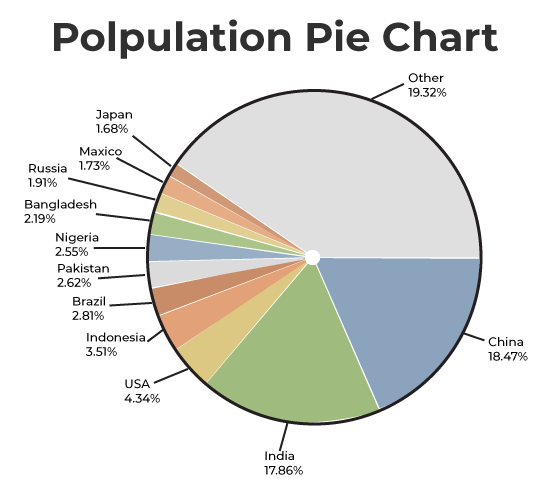Open in App
Not now

# Pie Chart

• Last Updated : 22 Sep, 2022

A pie chart or circle graph, in Data handling, is one type of pictorial representation of a given set of data, which is used to illustrate numerical problems by dividing a circular statistical graphic into slices or sectors. Here, the term “pie” corresponds to the word whole, while the term “sectors” or “slices” corresponds to parts of the whole. A pie chart formula is used to find the composition of something. The best real-life example of a circle graph or a pie chart is Pizza. Let us see the definition and pie chart example with proper explanation.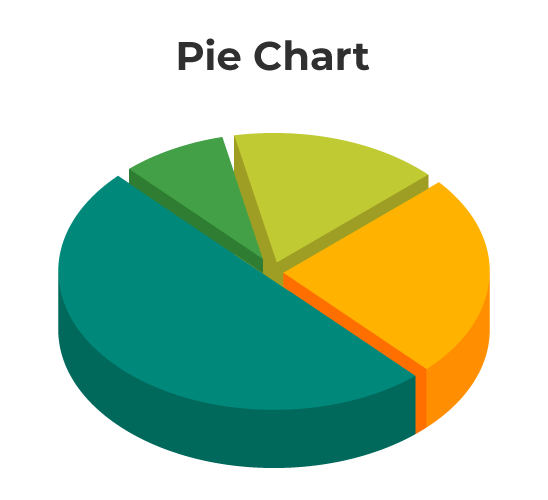## What is Pie Chart?

A pie chart is a pictorial or graphical representation of data in chart format. A pie chart uses a circle or sphere to represent the data, where the circle represents the entire data, and the slices represent the data in parts. Pie chart is one of the easiest ways to present and understand the given data, and pie charts are used very commonly. For example, pie charts are used in excel very often.

## Pie Chart Definition

There are different ways of data representation. A pie chart is one of the types of charts in which the data is represented in a circular shape. The pie chart circle is further divided into multiple sectors/slices; those sectors show the different parts of the data from the whole. Pie charts, also known as circle graphs or pie diagrams, are very useful in representing and interpreting data. The data can be compared easily with the help of a pie chart. Below is an example of a pie chart explained in detail.

## Pie Chart Examples

Let’s take a look at an example for a better understanding of pie charts. In a class of 200 students, a survey was done to collect each student’s favorite sports. The pie chart of the data is given below: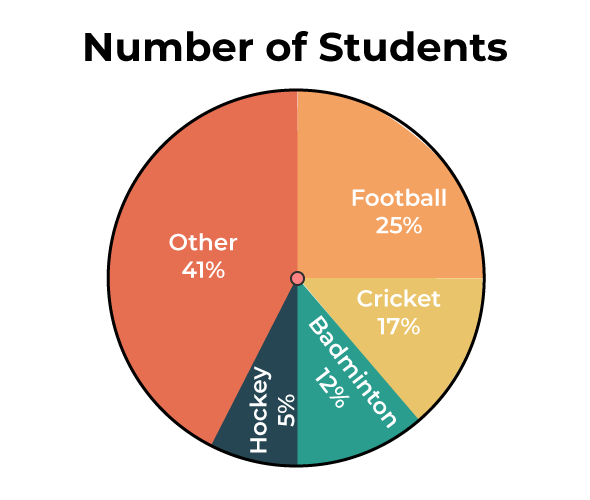Since the pie chart is provided and the total number of students is given, we can easily take the original data out for each sport.

• Cricket = 17/100 × 200 = 34 students
• Football = 25/100 × 200 = 50 students
• Badminton = 12/100 × 200 = 24 students
• Hockey = 5/100 × 200 = 10 students
• Other = 41/100 × 200 = 82 students

The original data for the pie chart shown above is given below:

## Pie Chart Formula

The total value or percentage of the pie is 100% always. Here it contains different sectors and segments in which each sector or segment of the chart corresponds to a certain portion of the net or total percentage (or data). The total or sum of all the data can be summed up to 360 degrees.

• Converting the data into degrees on a pie chart. The formula for a pie chart can be summed up as:

(Given Data / Total Value of Data) × 360°

• Calculating the percentage of each sector from degrees in a pie chart. To work out with degrees in a pie chart, we need to follow the following steps:
• At first, we need to measure every slice of the chart.
• Then we need to divide it by 360°.
• And finally, multiply the obtained result by 100.

The formula is given as:

(Frequency)/(Total Frequency) × 100

Calculating total number of sectors on a pie chart: To calculate the total number of slices or sectors on a pie chart, we need to multiply the sector’s percentage by the total value of the data and finally divide the result by 100. To work out the given percentage in a pie chart, we need to follow the following steps:

1. Categorize the data.
2. Then calculate the total.
3. Now we need to divide the categories.
4. After which, convert it into percentages.
5. And finally, calculate the degrees.

## Steps to Construct Pie Chart

We will learn how to create a pie chart step by step with the help of an example. A teacher surveyed a group of students to see what is their favorite hobby of each student. Let’s take a look at the pie chart example with an explanation. The data collected is listed as follows:

Now we will see how to construct a pie chart step by step.

Step 1: The first step requires us to jot down the available data in tabular form as follows:

Step 2: The next task is to calculate the sum of all the given data. Here, the Sum of All Data = (16 + 20 + 10 + 30 + 24) =100

Step 3: Now, the next task is to calculate the percentage of each sector. We need to divide each sector value by the sum or total and then multiply it by 100.

Step 4: Next step is to calculate the degrees corresponding to each slice. The values can be calculated as:

Central Angle of Each Component = (Given Data / Total Value of Data) × 360

Hence, The values are as follows:

Step 5: Now, with a protractor’s help, we will measure each angle from a single point or central point and draw the circle’s sectors. The resultant pie chart will be: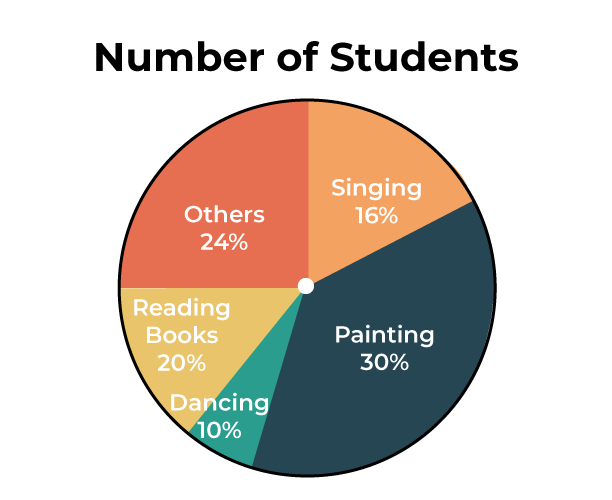## Interpreting Pie Chart

In order to interpret a pie chart, the first thing to notice is the data presented in the pie chart. If the data is given in percentage, it should be converted accordingly in order to analyze and interpret the data. Let’s take a look at an example in order to learn how to interpret pie charts.

Example: In a survey done among 300 people, it was observed which type of genre each person prefers. The pie chart of the same is mentioned below. Analyze and interpret the pie chart accordingly to find the original data.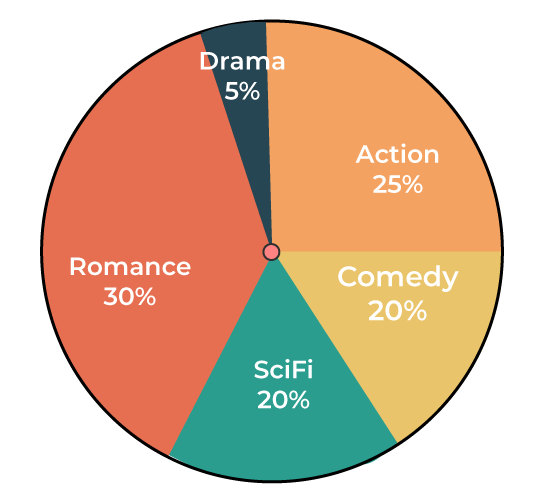Solution:

While observing the pie chart, it came to notice that the data is present in percentage. Lets convert the data to obtain the original value.

• Number of people who like comedy = 20/100 × 300 = 60 people.
• Number of people who like action = 25/100 × 300 = 75 people.
• Number of people who like romance = 30/100 × 300 = 90 people.
• Number of people who like drama = 5/100 × 300 = 15 people.
• Number of people who like sci-fi = 20/100 × 300 = 60 people.

Below given points are the advantages of the pie chart, which is the reason for its widespread application.

1. Pie chart is easily understood and comprehended.
2. Visual representation of data in a pie chart is done as a fractional part of a whole.
3. Pie chart provides an effective mode of communication to all types of audiences.
4. Pie chart provides a better comparison of data for the audience.

Pie charts have quite a few disadvantages as well. Below are the major disadvantages of the pie chart.

1. In the case of too much data, this presentation becomes less effective using a pie chart.
2. For multiple data sets, we need a series to compare them.
3. For analyzing and Assimilating the data in a pie chart, it is difficult for readers to comprehend.

## Uses of Pie Chart

Whenever a fraction or fractions are represented as a part of the whole, pie charts are used. Pie charts are used to compare the data and to analyze which data is bigger or smaller. Hence, while dealing with discrete data, pie charts are preferred. Let’s take a look at the uses of the pie chart:

• Pie charts are used to compare the profit and loss in businesses.
• In schools, the grades can be easily compared using a pie chart.
• The relative sizes of data can be compared using a pie chart.
• The marketing and sales data can be compared using a pie chart.

## Solved Examples on Pie Chart

Example 1: The given pie chart shows the subject of interest of each student in a class.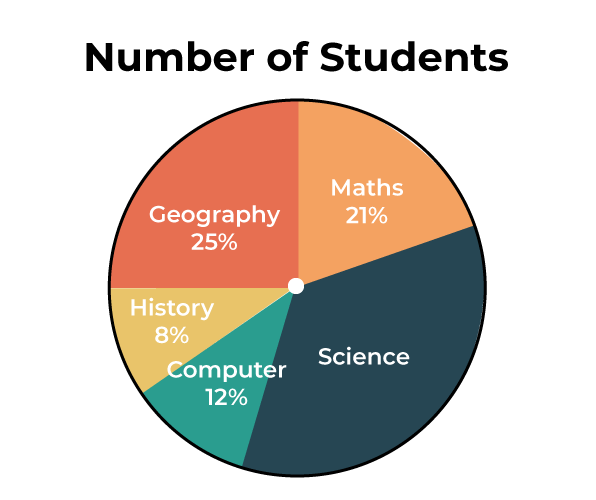Answer the following question concerning the given pie diagram.

1. If 30 students’ subjects of interest are history, how many total students were surveyed?
2. Which subject is liked the most?
3. Which subject is disliked the most?

Solution:

1. According to the given question, 8% of the total number of students is 30. i.e. (8/100) x Total = 30

Therefore, Total = 30 x (100/8) = 375

Hence 375 students were surveyed.

2. According to the given pie chart, science is liked the most.

3. According to the given pie chart, history is disliked the most.

Example 2: For a science camp, students from different states have enrolled. Construct a pie chart for the given table:

Solution:

Step 1: The first step requires us to jot down the available data into tabular form as follows:

Step 2: The next task is to calculate the sum of all the given data.

Here, the Sum of All Data = (10 + 5 + 5 + 10 + 10) = 40

Step 3: Now, the next task is to calculate the percentage of each sector. We need to divide each sector value by the sum or total and then multiply it by 100.

Step 4: Next step is to calculate the degrees corresponding to each slice. The values can be calculated as:

Step 5: Now, with a protractor’s help, we will measure each angle from a single point or central point and draw the circle’s sectors. The resultant pie chart will be: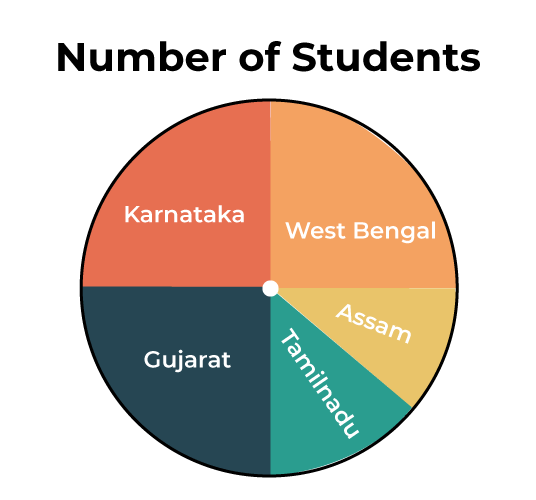Example 3: A pie chart is divided into four parts, and the values are given as x, 3x, 4x, and 4x. Find the value of x in degrees.

Solution:

As it is known that a pie chart has 360°. Therefore, if all the angles are added, it will give 360°.

x + 3x + 4x + 4x = 360°

12x = 360°

x = 30°

## FAQs on Pie Chart

Question 1: What is a pie chart?

Pie chart is the visual representation in which a circle graph is used to represent the values according to numbers, percentages, and degrees. The circle represents the entire data, and the sector or slices are used to represent values that are parts of the data.

Question 2: What is the formula for a pie chart?

To calculate the percentage of the given data, the formula used: (frequency ÷ total frequency) × 100.

Converting the data into degrees: (given data ÷ total data) × 100.

Question 3: How to create a pie chart?

Following are the steps that should be followed in order to create a pie chart:

Step 1: Add all values to find out the total data.

Step 2: In order to convert them in percentages, divide each value with the total data and multiply them with 100.

Step 3: Use the formula to find the degrees of each percentage value: (frequency ÷ total frequency) × 100.

Step 4: Now, draw a circle and using a protector, draw the sector based on the degree obtained w.r.t. the values.

Question 4: What are the examples of pie chart?

There are many examples of pie chart as pie chart is often used for visual representation. Following are some of the real-life examples:

• Representation of marks obtained by students.
• The marketing and sales data is obtained by using a pie chart.
• The profit and loss endured by a business can be represented by a pie chart.

Question 5: Should a pie chart be equal to 100?

Yes, a pie chart has a complete value of 100 and the values are represented in percentages w.r.t. 100.

Question 6: Generate world population by race pie chart 2021.